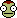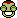# The Equation Game

## Recommended Posts

The object of the game is to somehow achieve the result of -100 or 100 with a series of equations, but each post may have only one equation. Please keep the equations simple, i.e. 25x1.8. Do not post equations like x times y = 99.628. If the result is achieved with less than five equations, it will be considered invalid. You may only erase the work of one-fourth of the other side in each equation. You may offer tips for solving your equation, but you must also post the answer/result, so the tips are mostly for if you think people might be confused by your equation even though it doesn't violate the rules.

Do not argue with people or pretend there is some sort of Number War. Do not have a specific positive and negative team.

Avoid doing anything that would upset the moderators.

Example:

Poster One: 4 times 9. This is 36.

Poster Two: 36 plus 4. This is 40.

Poster Three: 40 divided by 1.2. This is 33.33.

This would continue until -100 or 100 was reached.

I will start: 100 minus 100. This is 0 for fairness.

Edited by astrokerb

3.14 - .14.

This is 3.

3^3=27

27 x 0 = 0

0^0=1

?? 0° = O.

Now 1/0 =1i

##### Share on other sites

?? 0° = O.

Now 1/0 =1i

??? Is 1i supposed to be a variable or something?

Uh... Erm... 1+1+1+1+1+1+1+1+1+1=10? What just happened?

##### Share on other sites

38 minutes ago, max_creative said:

??? Is 1i supposed to be a variable or something?

Its up to your imaginationWhy 1/0 = 1i?

##### Share on other sites

Perhaps it's the only way his calculator can display a "divide by zero" error?

1i-i+23=24

Edited by Xorth Tanovar
##### Share on other sites

24 + 76 = 100, I win.

##### Share on other sites

1i is an imaginary number. From what I remember, an imaginary number can be attained when a number is divided by 0 or the square root of -1...

##### Share on other sites

Only sqrt(negative). Division by zero is to be resolved by L'Hospital rules.

##### Share on other sites

Don't "squirt negative" on this thread!0+1x1

##### Share on other sites

13 hours ago, kerbiloid said:

Only sqrt(negative). Division by zero is to be resolved by L'Hospital rules.

Hey, give me some slack...I haven't had calculus since 1987...## Join the conversation

You can post now and register later. If you have an account, sign in now to post with your account.
Note: Your post will require moderator approval before it will be visible.×   Pasted as rich text.   Paste as plain text instead

Only 75 emoji are allowed.